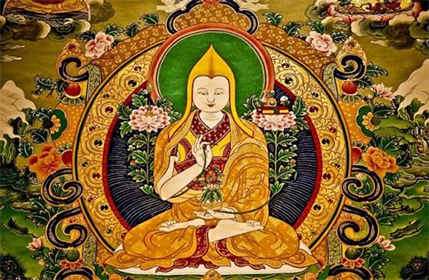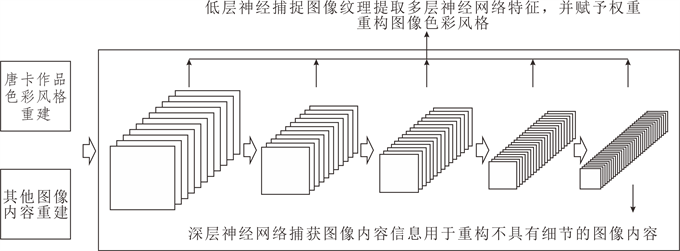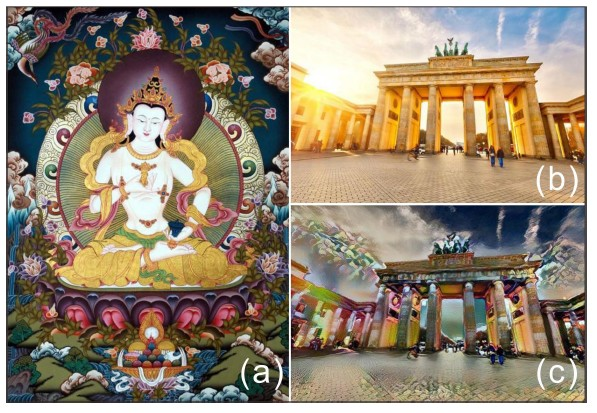# 基于深度神经网络的唐卡的色彩风格特征提取Color Style Feature Extraction of Thangka Based on Depth Neural Network

DOI: 10.12677/CSA.2019.911238, PDF, HTML, XML, 下载: 251  浏览: 509

Abstract: Thangka is an art of decorative painting with religious themes. It emphasizes contrast in color, pays attention to rich colors, and pursues resplendent effects. These brilliant colors give people visual impact and spiritual shock. Its original mission is to serve religion, draw materials from scriptures, and become exquisite works of art through exquisite painting. The content, color, shape and many other aspects of Thangka also provide a variety of reference for today’s development of painting art, but it is profound and difficult to copy. Image style transfer refers to the generation of style transfer map with both content and artistic style features by using the given content map and style map. The deep learning technology based on neural network promotes the research of image style transfer. This paper analyzes the color features of Thangka with the help of the depth neural network method which is developing rapidly in the field of vision, and extracts the color features of Thangka, which provides a new method for the research of the overall style transfer of Thangka. Using this method to conduct experiment on image data, this method can realize all style transfer of Thangka efficiently, quickly and naturally.

1. 引言Figure 1. Colorful Thangka

2. 深度神经网络Figure 2. Example of convolution kernel operationFigure 3. Image features change with network depth

3. 深度学习提取色彩特征方法

${\mathcal{L}}_{\text{content}}\left(\stackrel{\to }{p},\stackrel{\to }{x},l\right)=\frac{1}{2}{\sum }_{i,j}\left({F}_{ij}^{l}-{P}_{ij}^{l}\right)$ (1)

$\frac{\partial {\mathcal{L}}_{\text{content}}}{\partial {F}_{ij}^{l}}=\left\{\begin{array}{l}{\left({F}^{l}-{P}^{l}\right)}_{i,j}\text{\hspace{0.17em}}\text{\hspace{0.17em}}\text{\hspace{0.17em}}\text{\hspace{0.17em}}{F}_{ij}^{l}>0\\ 0\text{\hspace{0.17em}}\text{\hspace{0.17em}}\text{\hspace{0.17em}}\text{\hspace{0.17em}}\text{\hspace{0.17em}}\text{\hspace{0.17em}}\text{\hspace{0.17em}}\text{\hspace{0.17em}}\text{\hspace{0.17em}}\text{\hspace{0.17em}}\text{\hspace{0.17em}}\text{\hspace{0.17em}}\text{\hspace{0.17em}}\text{\hspace{0.17em}}\text{\hspace{0.17em}}\text{\hspace{0.17em}}\text{ }\text{\hspace{0.17em}}\text{ }{F}_{ij}^{l}<0\end{array}$ (2)

${G}_{ij}^{l}={\sum }_{k}{F}_{ik}^{l}{F}_{ik}^{l}$ (3)(4)

${\mathcal{L}}_{\text{style}}\left(\stackrel{\to }{a},\stackrel{\to }{x}\right)={\sum }_{l=0}^{L}{w}_{l}{E}_{l}$ (5)

$\frac{\partial {E}_{l}}{\partial {F}_{ij}^{l}}=\left\{\begin{array}{l}\frac{1}{{N}_{l}^{2}{M}_{l}^{2}}{\left({\left({F}^{l}\right)}^{\text{T}}\left({G}^{l}-{A}^{l}\right)\right)}_{ji}\text{\hspace{0.17em}}\text{\hspace{0.17em}}\text{\hspace{0.17em}}\text{\hspace{0.17em}}\text{\hspace{0.17em}}{F}_{ij}^{l}>0\\ 0\text{\hspace{0.17em}}\text{\hspace{0.17em}}\text{\hspace{0.17em}}\text{\hspace{0.17em}}\text{\hspace{0.17em}}\text{\hspace{0.17em}}\text{\hspace{0.17em}}\text{\hspace{0.17em}}\text{\hspace{0.17em}}\text{\hspace{0.17em}}\text{\hspace{0.17em}}\text{\hspace{0.17em}}\text{\hspace{0.17em}}\text{\hspace{0.17em}}\text{\hspace{0.17em}}\text{\hspace{0.17em}}\text{\hspace{0.17em}}\text{\hspace{0.17em}}\text{\hspace{0.17em}}\text{\hspace{0.17em}}\text{\hspace{0.17em}}\text{\hspace{0.17em}}\text{\hspace{0.17em}}\text{\hspace{0.17em}}\text{\hspace{0.17em}}\text{\hspace{0.17em}}\text{\hspace{0.17em}}\text{\hspace{0.17em}}\text{\hspace{0.17em}}\text{\hspace{0.17em}}\text{\hspace{0.17em}}\text{\hspace{0.17em}}\text{\hspace{0.17em}}\text{\hspace{0.17em}}\text{\hspace{0.17em}}\text{\hspace{0.17em}}\text{\hspace{0.17em}}\text{\hspace{0.17em}}\text{ }\text{ }{F}_{ij}^{l}<0\end{array}$ (6)

4. 唐卡风格特征迁移Figure 4. Examples of Thangka style extraction. (a) Thangka style extraction source image, (b) Input landscape image, (c) Thangka color feature landscape image

5. 结论

  冯骥才. 中国唐卡艺术集成, 玉树藏娘卷[M]. 出版地: 阳光出版社, 2010.  胡文瑾, 李战明, 刘仲民. 快速非局部均值形态成分分析唐卡图像修复算法[J]. 计算机辅助设计与图形学学报, 2014, 26(7): 1067-1074.  万德加. 唐卡色彩研究[D]: [硕士或博士学位论文]. 北京: 中央民族大学, 2017.  Lecun, Y., Bengio, Y. and Hin-ton, G. (2015) Deep Learning. Nature, 521, 436-444. https://doi.org/10.1038/nature14539  Gatys, L., Ecker, A., Bethge, M., et al. (2016) A Neural Algorithm of Ar-tistic Style. Journal of Vision, 16, 326. https://doi.org/10.1167/16.12.326  Wang, X., Tao, Q., Wang, L., et al. (2015) Deep Convolutional Architecture for Natural Image Denoising. International Conference on Wireless Communications & Signal Processing, Nanjing, 15-17 October 2015, 1-4. https://doi.org/10.1109/WCSP.2015.7341021